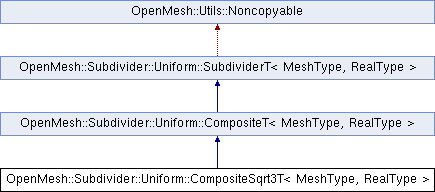Developer Documentation
OpenMesh::Subdivider::Uniform::CompositeSqrt3T< MeshType, RealType > Class Template Reference

`#include <libs_required/OpenMesh/src/OpenMesh/Tools/Subdivider/Uniform/CompositeSqrt3T.hh>`

Inheritance diagram for OpenMesh::Subdivider::Uniform::CompositeSqrt3T< MeshType, RealType >:struct  FVCoeff

## Public Types

typedef CompositeT< MeshType, RealType > InheritedPublic Types inherited from OpenMesh::Subdivider::Uniform::CompositeT< MeshType, RealType >
typedef RealType real_t

typedef MeshType mesh_t

typedef SubdividerT< mesh_t, real_t > parent_tPublic Types inherited from OpenMesh::Subdivider::Uniform::SubdividerT< MeshType, RealType >
typedef MeshType mesh_t

typedef RealType real_t

## Public Member Functions

CompositeSqrt3T (MeshType &_mesh)

const char * name () const
Return name of subdivision algorithm.Public Member Functions inherited from OpenMesh::Subdivider::Uniform::CompositeT< MeshType, RealType >
CompositeT (MeshType &_mesh)Public Member Functions inherited from OpenMesh::Subdivider::Uniform::SubdividerT< MeshType, RealType >
virtual ~SubdividerT ()
Descructor (calls detach())

SubdividerT (void)

SubdividerT (MeshType &_m)

bool operator() (MeshType &_m, size_t _n, const bool _update_points=true)

bool attach (MeshType &_m)

bool operator() (size_t _n, const bool _update_points=true)

void detach (void)

## Protected Types

typedef Inherited::Coeff CoeffProtected Types inherited from OpenMesh::Subdivider::Uniform::CompositeT< MeshType, RealType >
typedef MeshType::Scalar scalar_t

typedef MeshType::VertexHandle VertexHandle

typedef MeshType::FaceHandle FaceHandle

typedef MeshType::EdgeHandle EdgeHandle

typedef MeshType::HalfedgeHandle HalfedgeHandle

## Protected Member Functions

void apply_rules (void)Protected Member Functions inherited from OpenMesh::Subdivider::Uniform::CompositeT< MeshType, RealType >
bool prepare (MeshType &_m)

bool subdivide (MeshType &_m, size_t _n, const bool _update_points=true)
Subdivide mesh `_m` `_n` times.

bool cleanup (MeshType &_m)
Cleanup mesh after usage, e.g. remove added properties.

void commit (MeshType &_m)

void corner_cutting (HalfedgeHandle _heh)
Corner Cutting.

VertexHandle split_edge (HalfedgeHandle _heh)
Split Edge.

void Tvv3 ()
Split Face, using Vertex information (1-3 split)

void Tvv4 ()
Split Face, using Vertex information (1-4 split)

void Tfv ()
Split Face, using Face Information.

void FF ()
Face to face averaging.

void FFc (Coeff &_coeff)
Weighted face to face averaging.

void FFc (scalar_t _c)
Weighted face to face averaging.

void FV ()
Face to vertex averaging.

void FVc (Coeff &_coeff)
Weighted face to vertex Averaging with flaps.

void FVc (scalar_t _c)
Weighted face to vertex Averaging with flaps.

void FE ()
Face to edge averaging.

void VF ()
Vertex to Face Averaging.

void VFa (Coeff &_coeff)
Vertex to Face Averaging, weighted.

void VFa (scalar_t _alpha)
Vertex to Face Averaging, weighted.

void VV ()
Vertex to vertex averaging.

void VVc (Coeff &_coeff)
Vertex to vertex averaging, weighted.

void VVc (scalar_t _c)
Vertex to vertex averaging, weighted.

void VE ()
VE Step (Vertex to Edge Averaging)

void VdE ()
Vertex to edge averaging, using diamond of edges.

void VdEc (scalar_t _c)
Weighted vertex to edge averaging, using diamond of edges.

void VdEg (Coeff &_coeff)

void VdEg (scalar_t _gamma)

void EF ()
Edge to face averaging.

void EV ()
Edge to vertex averaging.

void EVc (Coeff &_coeff)
Weighted edge to vertex averaging.

void EVc (scalar_t _c)
Weighted edge to vertex averaging.

void EdE ()
Edge to edge averaging w/ flap rule.

void EdEc (scalar_t _c)
Weighted edge to edge averaging w/ flap rule.

## Protected Attributes

OpenMesh::Subdivider::Uniform::CompositeSqrt3T::FVCoeff coeffs_

## Detailed Description

### template<typename MeshType, typename RealType = float> class OpenMesh::Subdivider::Uniform::CompositeSqrt3T< MeshType, RealType >

Uniform composite sqrt(3) subdivision algorithm

Definition at line 81 of file CompositeSqrt3T.hh.

## Member Function Documentation

template<typename MeshType , typename RealType = float>
 void OpenMesh::Subdivider::Uniform::CompositeSqrt3T< MeshType, RealType >::apply_rules ( void )
inlineprotectedvirtual

Assemble here the rule sequence, by calling the constructor of the wanted rules.

Definition at line 99 of file CompositeSqrt3T.hh.

The documentation for this class was generated from the following file: